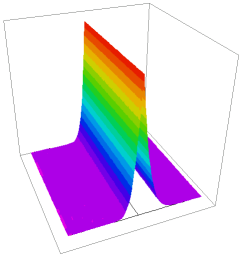﻿ Sample Problems > Usage > Contact_Boundaries > transient_contact_resistance_heating

# transient_contact_resistance_heating

Navigation:  Sample Problems > Usage > Contact_Boundaries >

# transient_contact_resistance_heating{ TRANSIENT_CONTACT_RESISTANCE_HEATING.PDE

This is a time-dependent version of the example CONTACT_RESISTANCE_HEATING.PDE

An electrical current passes through a material with an electrical contact resistance

on the center plane.  The resistance heating at the contact drives a time-dependent

heat equation.

 }   title "transient contact resistance heating"   variables    V    Temp(0.001)   definitions    Kt             { thermal conductivity }    Heat  =0    Rc = 2         { Electrical contact resistance }    rho = 1         { bulk resistivity }    sigma = 1/rho   { bulk conductivity, I=sigma*grad(V) }   Initial values    V = x/3     { a reasonable guess }    Temp = 0   equations    V:       div(sigma*grad(V))  = 0    Temp:    div(Kt*grad(Temp)) + Heat = dt(Temp)   boundaries     Region 1        Kt=15       start (0,0)natural(V)=0   natural(temp)=0     line to (3,0)

value(V)=1     value(temp)=0       line to (3,3)

natural(V)=0   natural(temp)=0     line to (0,3)

value(V)=0     value(temp)=0       line to close

Region 2

Kt=5

start (0,0)     line to (1.5,0)

contact(V) = (1/rc)*JUMP(V)     { resistance jump }

natural(temp) = JUMP(V)^2/Rc   { heat generation }

line to(1.5,3)

natural(V)=0 natural(Temp)=0

line to (0,3) to close

time 0 to 5 by 1e-6

monitors

for cycle=5

contour(Temp)

plots

for cycle=20

grid(x,y)

contour(V)    painted

contour(Temp)    painted

surface(Temp)

contour(kt*dx(temp))    painted

contour(kt*dx(temp))    painted

elevation(V) from(0,1.5) to (3,1.5)

elevation(temp) from(0,1.5) to (3,1.5)

elevation(dx(v)) from(0,1.5) to (3,1.5)

elevation(kt*dx(temp)) from(0,1.5) to (3,1.5)

histories

history(Temp) at (0.5,1.5) (1.0,1.5) (1.5,1.5) (2.0,1.5) (2.5,1.5)

end